## Monday, 3 January 2011

### Another Go-Round with Archimedes

I just flew back to the UK from the US, and had time to read some translations from Archimedes' "On the Sphere and Cylinder."

The sad truth is that reading Archimedes always makes me feel a little stupid. It is not just that he was a genius, but that each time I read him, I realize some simple fact that I ought to have somehow noticed years before.

First some background notes: I wrote a while back about the fact that Archimedes discovered that the surface area of a sphere, between any two parallel cutting planes is exactly the same as the surface area of the cylinder with a diameter equal to the diameter of the sphere and a height equal to the distance between the two planes.On this pass I noticed that he had given a very simple answer to a question that seems harder: What is the surface area of the segment of a sphere which has a height of h and a radius of the base is a. (see image)The image gives a hint to how I would solve it. Use the given information to find the radius of the sphere, then use the fact that the surface of the segment is the same as the lateral surface of a cylinder with a diameter of 2r and a height of h, that is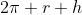If we followed up on this, we see that r2 = a2 + (r-h)2
If we expand that we get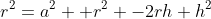and subtracting r^2 from both sides gives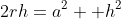and solving for r we get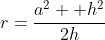.. Now if we plug that in for the r inwe get an area of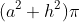. As Archimedes so cleverly determined, the area is independent of the sphere it was situated on. The area is the same as a circle whose radius is the distance from the vertex of the segment to a point on the circular base.

Wait, there is a little more. When I wrote about isoperimetric problems when bound against an edge (find the largest rectangular area that can be enclosed by n ft of fence built with a barn as one edge of the pen) I never thought about a simple 3d example of this same idea.... but Archimedes did. He observed that of all the spherical segments with a given surface area, the largest volume was for the one that enclosed a hemisphere. Very Clever.... another note about an "aha" moment in a future blog.